# Quickly Get Sheet Name

This post explains that how to get the sheet name only in your current worksheet in excel. How do I insert the current worksheet’s name into one cell quickly.

## Get Sheet Name with Formula

If you want to quickly get current worksheet name only, then inert it into one cell. You can use a formula based on the MID function in combination with the FIND function and the Cell function.

You can type the following formula into the formula box of cell B1, then press enter key.

`=MID(CELL("filename",B1),FIND("]",CELL("filename",B1))+1,255)`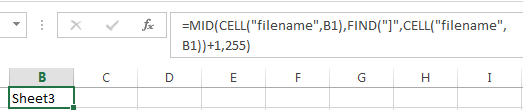Let’s see how this formula works:

=CELL(“filename”,B1)

The cell function will get the full file name and its path of your current worksheet. The result look like this: C:\ [book1.xlsm]sheet1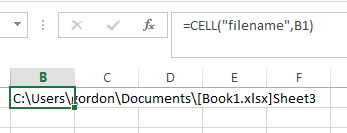=FIND(“]”,CELL(“filename”,B1))+1

The FIND function will get the first position of the character “]”, then passed it into the MID function to extract the sheet name.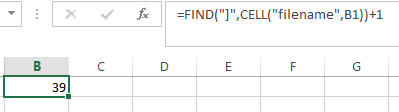## Get Sheet Name with VBA

You can also use an Excel VBA Macro to get all worksheet’s name and insert those sheet name into the different cells. Just do the following steps:

#1 click on “Visual Basic” command under DEVELOPER Tab.#2 then the “Visual Basic Editor” window will appear.

#3 click “Insert” ->”Module” to create a new module.#4 paste the below VBA code into the code window. Then clicking “Save” button.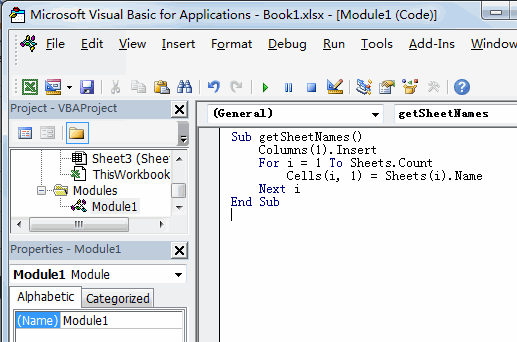#5 back to the current worksheet, then run the above excel macro. Click Run button.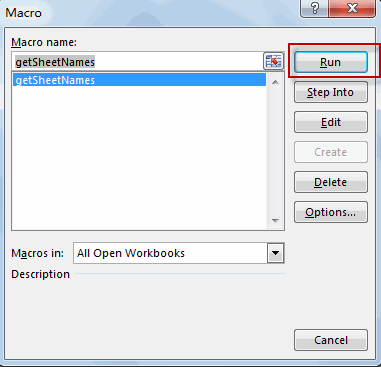#6 Lets see the result: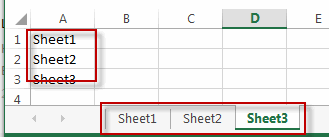### Related Functions

• Excel FIND function
The Excel FIND function returns the position of the first text string (sub string) within another text string.The syntax of the FIND function is as below:= FIND(find_text, within_text,[start_num])…
• Excel MID function
The Excel MID function returns a substring from a text string at the position that you specify.The syntax of the MID function is as below:= MID (text, start_num, num_chars)….
• Excel CELL function
The Excel CELL function returns information about the formatting, location, size, or contents of a cell.The syntax of the CELL function is as below:= CELL (info_type,[reference])…